UPSC  >  Examples: Probability

# Examples: Probability Notes | Study CSAT Preparation for UPSC CSE - UPSC

## Document Description: Examples: Probability for UPSC 2022 is part of CSAT Preparation for UPSC CSE preparation. The notes and questions for Examples: Probability have been prepared according to the UPSC exam syllabus. Information about Examples: Probability covers topics like and Examples: Probability Example, for UPSC 2022 Exam. Find important definitions, questions, notes, meanings, examples, exercises and tests below for Examples: Probability.

Introduction of Examples: Probability in English is available as part of our CSAT Preparation for UPSC CSE for UPSC & Examples: Probability in Hindi for CSAT Preparation for UPSC CSE course. Download more important topics related with notes, lectures and mock test series for UPSC Exam by signing up for free. UPSC: Examples: Probability Notes | Study CSAT Preparation for UPSC CSE - UPSC
 1 Crore+ students have signed up on EduRev. Have you?

CAT exam is all about how smartly you solve the question in the quickest way. To help you with that, we at EduRev have started a summary sheet of formulae which will have all the shortcuts & formulae in one sheet. In this document you would be revising all the important parts of Probability, this document helps you in understanding the shortcuts & formulae of probability in a faster way.

Important Tips & Formulae of Probability
The probability of an event A is the number of ways event A can occur divided by the total number of possible outcomes. The probability of an event A, symbolized by P(A), is a number between 0 and 1, inclusive, that measures the likelihood of an event in the following way:

• If P(A) > P(B) then event A is more likely to occur than event B.
• If P(A) = P(B) then events A and B are equally likely to occur.
• Two events are mutually exclusive if the intersection of two events is null (A ∩ B = φ)
• If E and F two events then P(E U F) = P(E) + P(F) - P(E and F together)
• If an event E is sure to occur, you say that the probability of event E is equal to 1 and you write P (E) = 1. Such events are known as certain events.
• If an event E is sure not to occur, you say that the probability of the Event E is equal to 0 and you write P(E) = 0. Such events are known as impossible events.
• The probability of E not occurring, denoted by P(not E), is given by P(not E) or P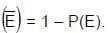• The odds in favor of occurrence of the event A are defined by m: n – m i.e., P (A): P (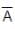) and the odds against the occurrence of A are defined by n – m : m, i.e., P (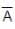): P (A)
• To find the probability of two or more independent events occurring in sequence, find the probability of each event occurring separately, and then multiply the answers.

Example:  If a number is selected at random from the 2 digit 6 multiples, what is the probability that it is divisible by 9?
Solution. Total 6 multiples = 12, - - - -, 96 ⇒ 15.
Required numbers are both multiples of 6 & 9
i. e, the multiples of 18. Those are 18, 36,- - - -, 90, total 5.
∴ Ans =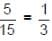Practice Question

Question for Examples: Probability
Try yourself:Out of 13 applicants for a job, there are 5 women and 8 men. Two persons are to be selected for the job. The probability that at least one of the selected persons will be a woman is:

Example: If 2 dies are thrown, what is the probability of getting both the numbers prime?
Solution: P (Prime on first die) × P(Prime on second die)
=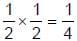Example: If 3 dies are thrown, what is the probability of getting a sum 4.
Solution: The possibility is 1, 1, 2 or 1, 2, 1 or 2, 1, 1.
∴ Ans =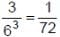Example: If 2 cards are drawn from a pack, what is the probability that both the cards are of different colours?
Solution: The possibility is 1 black and 1 Red.
i.e,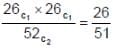Example: There are 5 red, 4 green, 3 yellow & 8 white balls in a bag, If three balls are chosen at random without replacement, what is the probability that they are of same color.
Solution: All the three can be red or green or yellow or white.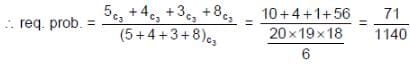Example: In a game, there are three rounds; the probabilities of winning first, second & third rounds are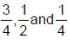respectively. A prize will be given, if any one wins all the rounds. What is the probability of winning the prize?
Solution: All the rounds have to be won to get the prize. So, the required answer is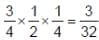.

The document Examples: Probability Notes | Study CSAT Preparation for UPSC CSE - UPSC is a part of the UPSC Course CSAT Preparation for UPSC CSE.
All you need of UPSC at this link: UPSC

## CSAT Preparation for UPSC CSE

72 videos|64 docs|92 tests
 Use Code STAYHOME200 and get INR 200 additional OFF

## CSAT Preparation for UPSC CSE

72 videos|64 docs|92 tests

### How to Prepare for UPSC

Read our guide to prepare for UPSC which is created by Toppers & the best Teachers

Track your progress, build streaks, highlight & save important lessons and more!

,

,

,

,

,

,

,

,

,

,

,

,

,

,

,

,

,

,

,

,

,

;